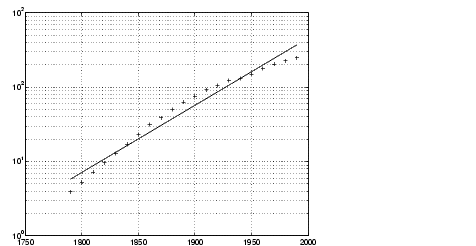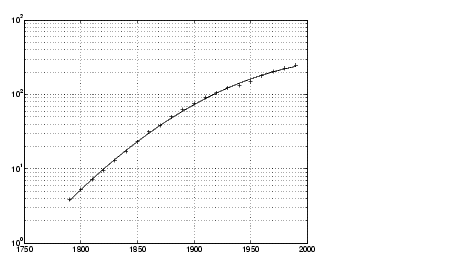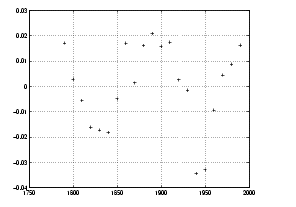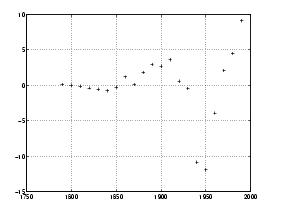MathematicsExponential Fit

By looking at the population data plots on the previous pages, the population data curve is somewhat exponential in appearance. To take advantage of this, let's try to fit the logarithm of the population values, again working with normalized year values.

• ```logp1 = polyfit(sdate,`log10`(pop),1);
logpred1 = 10.^polyval(logp1,sdate);
`semilogy`(cdate,logpred1,'-',cdate,pop,'+');
grid on```

Now try the logarithm analysis with a second-order model.

• ```logp2 = polyfit(sdate,log10(pop),2);
logpred2 = 10.^polyval(logp2,sdate);
semilogy(cdate,logpred2,'-',cdate,pop,'+'); grid on```

This is a more accurate model. The upper end of the plot appears to taper off, while the polynomial fits in the previous section continue, concave up, to infinity.

Compare the residuals for the second-order logarithmic model.

 Residuals in Log Population Scale Residuals in Population Scale `logres2 = log10(pop)- polyval(logp2,sdate);``plot(cdate,logres2,'+')``r = pop - 10.^(polyval(logp2,sdate));``plot(cdate,r,'+')`The residuals are more random than for the simple polynomial fit. As might be expected, the residuals tend to get larger in magnitude as the population increases. But overall, the logarithmic model provides a more accurate fit to the population data.Analyzing Residuals Error Bounds© 1994-2005 The MathWorks, Inc.